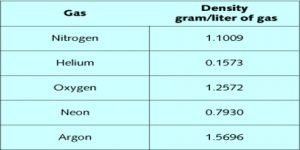# Density of Gases in Molecular Mass

Density of Gases in Molecular Mass

The density of a gas is defined in Physical Chemistry as the mass in g of 1 L of gas. As the volume depends on the pressure and temperature these must also be specified while making statement about density. Normal density of a gas is the mass of 1 L of gas measured at 00 C and l atm pressure, i.e., at S.T.P. Under ordinary conditions gases are about 1/100 times as dense as liquids. Thus 1 mL of water at 4°C weighs 1 g, whereas the same volume of air weighs only about 0.0013 g. It is, therefore, evident that the experimental determination of densities is difficult and the methods used have necessarily to be delicate if good results are to be obtained.Densities of gases are useful in calculating molecular masses. The ideal gas law equation is used for the purpose. We have seen that….

PV = nRT = g/M RT

From this one can readily deduce that,

P = ρ . (RT/M)

where ρ is the density of the gas. In using this equation for obtaining molecular mass, the value of R used will depend on the unit in which P and V are expressed. Thus if pressure is in atmosphere and volume in L, R is to he expressed in L-atm units.

If a liquid can be conveniently converted into the vapour state, the measurement of the density of vapour will yield the molecular mass of the liquid in the vapour state. The molecular masses of gases and vapours will be approximate as the equation from which the molecular mass is derived is not strictly obeyed by real gases. The following methods are used for the determination of gas density.

(i) Regnault’s method,

(ii) Dumas method,

(iii) Hofmann’s method,

(iv) Victor Meyer’s method,

(v) the buoyancy method and

(vi) the method of limiting density.